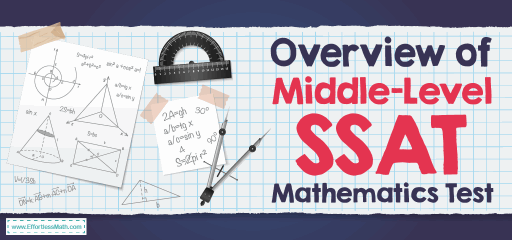# Overview of Middle-Level SSAT Mathematics TestThe Secondary Schools Admission Test is as known as SSAT is a test used to help determine admission to private elementary, middle, and high schools. If you want to attend an elite private middle or high school, this is a test you should do well. The Students take the test based on their current grade, not the one to which they’ll be applying. For example, if you are in grade 7, you can take the middle-level test, not the upper-level one.

The Middle-Level Secondary School Admission Test (SSAT) is a multiple-choice test for students currently in grades 5-7 that includes quantitative (math), verbal, and reading comprehension sections. The writing section, although still without points, is provided at the beginning of the test. It also has a non-scoring experimental section with 16 multiple-choice questions. It asks for six verbal, five readings, and five math questions to assess content for future exams.

There are 167 items in this test, but only 150 points to earn your score. The total test time of this test is 3 hours and 5 minutes. This includes a 5-minute break and a 10-minute break.

## How is the structure of Middle-Level SSAT Mathematics?

The Middle-Level SSAT Mathematics section is divided into two parts. Each part has 25 questions, and you have 30 minutes for each section. This test measures your ability to solve problems involving elementary algebra, arithmetic, geometry, and other concepts. These topics involve:

### Number concepts and operations:

This involves subtraction, addition, multiplication, division, ratios, percentages, arithmetic word problems, estimation, rational numbers, sequences, and frequencies.

### Algebra:

This includes algebraic word problems, patterns, line equations, absolute value, and exponents.

### Geometry/measurement:

The Geometry/measurement asks about the area and circumference of circles, area, and perimeter of polygons, properties of triangles, volume, and parallel and coordinate geometry, perpendicular lines, and slope.

### Data analysis/probability:

This involves interpreting tables and graphs, trends, inferences, and probability.

Many of the questions that appear in the Middle-Level SSAT mathematics are structured mathematically and directly state what you need to do; others may not. In these problems, carefully consider how to provide information and how to ask questions to determine the operation (s) to be performed.

## Is the Middle-Level SSAT Mathematics test hard?

The Middle-Level SSAT is middle difficult to design, and like any other test, it can be stressful for middle school students. The Middle-Level SSAT mathematics test is especially challenging for many students.

## Can you use a calculator on the Middle-Level SSAT Mathematics?

The Students are NOT allowed to use a calculator on the Middle-Level SSAT mathematics.

## How is theMiddle-Level SSAT test scored?

In Middle-Level SSAT, students receive 1 point for correct answers, lose ¼ point for wrong questions, and 0 points for unanswered questions.

The lowest number on the scale (440) is the lowest score a student can achieve, and the highest number (710) is the highest score for each section. The total scaled score for the SSAT Middle-Level Test is the sum of the scores of the verbal, quantitative, and reading sections. Its low value is 1320, high value 2130, and midpoint 1725.

## The Best Books to Ace the SSAT Middle-Level MathTest

### What people say about "Overview of Middle-Level SSAT Mathematics Test - Effortless Math: We Help Students Learn to LOVE Mathematics"?

No one replied yet.

X
23% OFF

Limited time only!

Save Over 23%

SAVE \$5

It was \$21.99 now it is \$16.99